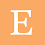# What is Algorithms and its Characteristics with examples

Algorithm:-
• An algorithm is a finite lists of instructions in sequence to solve the computation problems.
• An algorithm is a step by step of finite number of process to solve the problems.You can write the algorithms in any language which is understandable to the persons (programmers)
• In Real life,an algorithm is a recipe for any cooking dish.
Characteristics of Algorithms:-
There are following characteristics of any algorithms as given below.
1. Input:-An algorithm should have one or more inputs.
2. Output:-An algorithm must have at least one output.
3. Definiteness:- Every statement in any algorithm should be definiteness.It means.Every statement in algorithm should have unambiguous.Every statement should have clear and there should not be more than one way to interprate the single statement in given algorithm.
4. Finiteness:-An algorithm should have a finite number of steps(instructions) to solve the problems and get a valid output.
5. Effectiveness:-An algorithm should have effectiveness and produce well defined output of any programs. Effectiveness means, an algorithms should have good method which produce effective output with less time and less storage capacity.
Design of Algorithms:-There are different ways to represent an algorithm as given below:-
1. Natural Language:- We can write the algorithms in any natural language which is understandable to the persons..Natural language is a human language. eg. English, Hindi ,French ,Chinese  etc.Natural languages are not a effective language.It is a ambiguous language.It doesn't give a precise idea .
2. Pseudo code:-Pseudo codes gives precise idea for any algorithms.It is unambiguous in nature.There are many control statements like for ,if,while,do,etc.It gives iterative codes that makes an algorithm effectiveness.We are generally use this language to write the precise codes.
3. Flow chart:-This another way to write an algorithm to solve step by step problems in graphical manner.We will learn this technique in details in our coming lectures.
Different between Algorithms and programs:-
There are some difference between algorithms and programs as given below:-
1. Start
3. Scan n elements in array A
4. Declare a  sum variable
5. Assign zero value in sum variable
6. Add all array elements using for loop
7. Display the sum
8. stop
#Write a Algorithm in Pseudo code:-
1. start
3. sum<--0
4. for <--1 to n do
5. sum<--sum A[i]
6. display sum
7. stop
#Write a Algorithm in Flow Chart:-
Python Programs to add an Array elements:-
``````#intialize the array
A=[1,2,3,5,8,9,11,13,15,17]
sum=0
for i in range(0,len(A)):
sum=sum+A[i]
print("Sum of all array elements is=",sum)
``````
Output of Above Python Codes:-
Sum of all array elements is= 84

For More...
Watch Lecture 18 complete Video

#### 1 comment:

1.